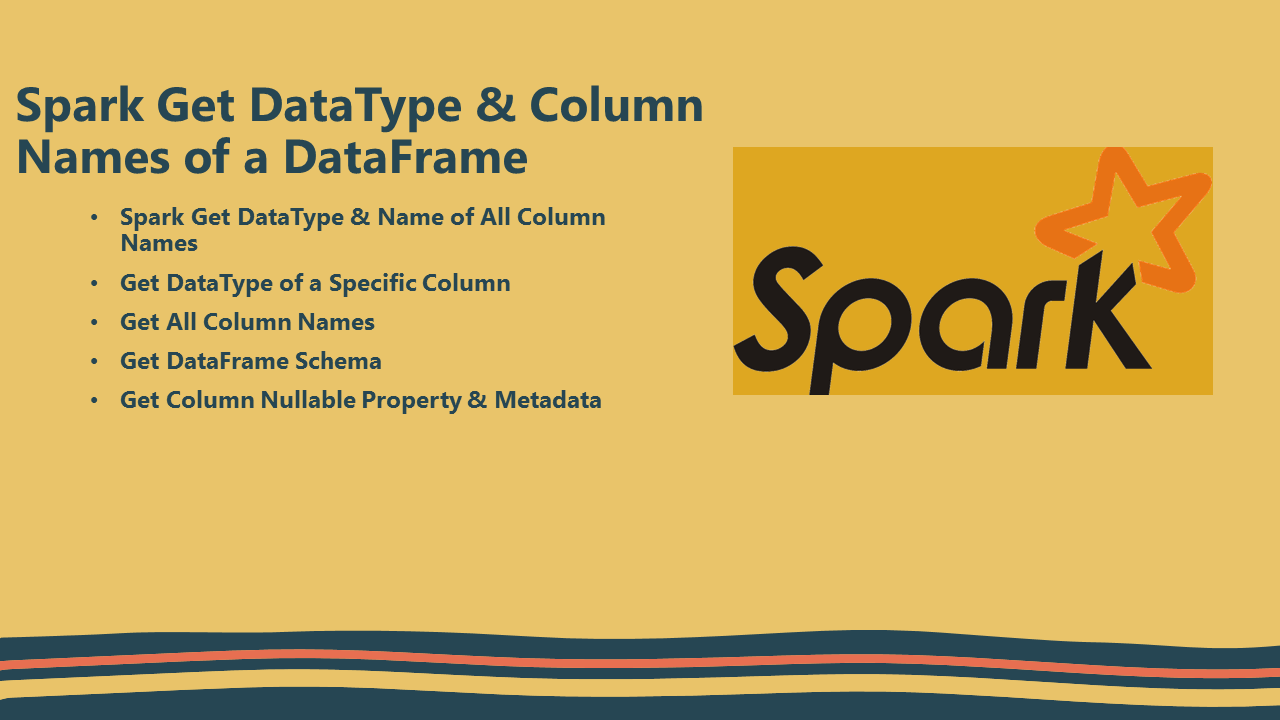# Spark Get DataType & Column Names of DataFrame

In Spark you can get all DataFrame column names and types (DataType) by using `df.dttypes` and `df.schema` where `df` is an object of DataFrame. Let’s see some examples of how to get data type and column name of all columns and data type of selected column by name using Scala examples.

## 1. Spark Get All DataType & Column Names

First, let’s see how to get all data types (DataType) & column names using `df.dttypes`; where `dttypes` returns all Spark DataFrame columns as `Array[(String,Stirng)]`. The first value in the tuple is the column name and the second value is a data type.

``````
import spark.sqlContext.implicits._
val df = Seq((1,"Robert"), (2,"Julia")).toDF("id","name")

// Get All column names and it's types
df.dtypes.foreach(f=>println(f._1+","+f._2))

// Prints below column name & data type
// Id,IntegerType
// Name,StringType
``````

Similarly, you can also get the data type & name of all columns using `df.schema`, `schema` returns a StructType which is an array of StructField, and by using its methods you can get the column name and its type. `df.schema.fields` returns `Array[StructField]`.

``````
// Get All column names and it's types
df.schema.fields.foreach(f=>f.name +","+f.dataType)
``````

This yields the same output as above.

## 2. Get DataType of a Specific Column Name

If you want to get the data type of a specific DataFrame column by name then use the below example.

``````
// Get data type of a specific column
println(df.schema("name").dataType)

// Prints data type of a "name" column
// StringType
``````

## 3. Get All Column Names

You can get the all columns of a Spark DataFrame by using `df.columns`, it returns an array of column names as `Array[Stirng]`.

``````
// Get All column names from DataFrame
val allColumnNames=df.columns
println(allColumnNames.mkString(","))

// Print all column names in comma separated string
// Id,name
``````

## 4. Get DataFrame Schema

As you would already know, use `df.printSchama()` to display column name and types to console, similarly d`f.schema.printTreeString()` also prints schema to console.

``````
df.printSchema()

// root
// |-- id: integer (nullable = false)
// |-- name: string (nullable = true)

``````

## 5. Get Column Nullable Property & Metadata

Let’s see how to get if a column is accepts null values (Nullable) and Metadata of the column.

``````
// Get Column Nullable Property & Metadata
print(df.schema("name").nullable)
``````

## 6. Other ways to get DataFrame Schema

``````
print(df.schema.toDDL)
// `id` INT,`name` STRING

print(df.schema.prettyJson)
//{
//  "type" : "struct",
//  "fields" : [ {
//    "name" : "id",
//    "type" : "integer",
//    "nullable" : false,
//  }, {
//    "name" : "name",
//    "type" : "string",
//    "nullable" : true,
//  } ]
// }
``````

## 7. Conclusion

In summary, you can get the names and data type’s (DataType) of all DataFrame column’s by using `df.dttypes` and `df.schema` and also you can use several StructFeild methods to get the additional details of the Spark DataFrame column.

Happy Learning !!

### Naveen (NNK)

Naveen (NNK) is a Data Engineer with 20+ years of experience in transforming data into actionable insights. Over the years, He has honed his expertise in designing, implementing, and maintaining data pipelines with frameworks like Apache Spark, PySpark, Pandas, R, Hive and Machine Learning. Naveen journey in the field of data engineering has been a continuous learning, innovation, and a strong commitment to data integrity. In this blog, he shares his experiences with the data as he come across. Follow Naveen @ @ LinkedIn